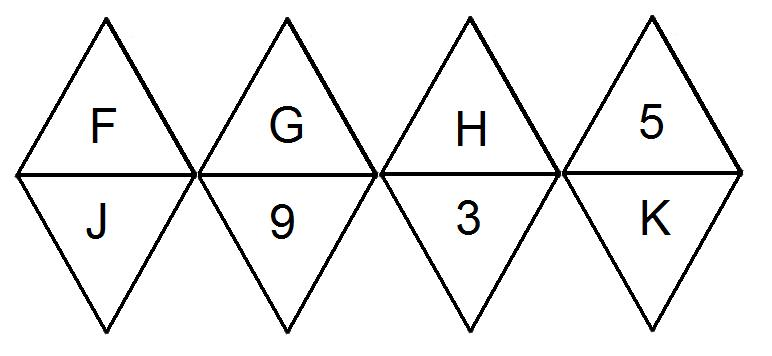#### You may also like### Consecutive Numbers

An investigation involving adding and subtracting sets of consecutive numbers. Lots to find out, lots to explore.### Calendar Capers

Choose any three by three square of dates on a calendar page...### Days and Dates

Investigate how you can work out what day of the week your birthday will be on next year, and the year after...

# Magic Octahedron

##### Age 11 to 14 ShortChallenge Level

The diagram show the net of a regular octahedron.In a magic octahedron, the four numbers on the faces that meet at a vertex add up to make the same total for every vertex. If the letters $F, G, H, J$ and $K$ are replaced with the numbers $2$, $4$, $6$, $8$, and some other number, in some order, to make a magic octahedron, what is the value of $G + J$?

If you liked this problem, here is an NRICH task which challenges you to use similar mathematical ideas.

This problem is taken from the UKMT Mathematical Challenges.
You can find more short problems, arranged by curriculum topic, in our short problems collection.